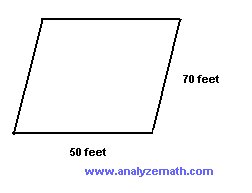# Perimeter - Grade 5 Maths Questions With Solutions and Explanations

Solutions and explanations to perimeter - grade 5 questions are presented.

 Figure B is inside figure A shown below. Which statement is correct?The perimeter of A is equal to the perimeter of B The perimeter of A is smaller than the perimeter of B The perimeter of A is larger than the perimeter of B cannot answer the question Solution Only statement C "The perimeter of A is larger than the perimeter of B" is correct since figure B is inside figure A and therefore its perimeter is smaller than that of A. Find the perimeter of the rectangle shown below.Solution The perimeter of a rectangle is equal to twice its length plus twice its width. The perimeter of the given rectangle is equal to 2 � 12 + 2 � 9= 24 + 18 = 42 cm Find the perimeter of the square shown below.Solution The perimeter of the square is equal to 15 + 15 + 15 + 15 = 4 � 15 = 60 cm Find the perimeter of the shape below.Solution We first find the missing sides (in red) in the given figure.Right side = 12 - 7 = 5 mm Bottom side = 15 + 8 = 23 mm We now calculate the perimeter P by adding all sides of the given shape starting from top left P = 15 + 7 + 8 + 5 + 23 + 12 = 70 mm What is the perimeter of the parallelogram shown below?Solution A parallelogram has opposite sides equal in length. The perimeter is found by adding all 4 sides of the of the parallelogram. The perimeter is given by 50 + 70 + 50 + 70 = 2 � 50 + 2 � 70 = 240 feet What is the perimeter of a an equilateral triangle of side 20 cm? Solution An equilateral triangle has all 3 sides equal. It perimeter is 3 times one side and is equal to 3 � 20 = 60 cm A plane figure has 5 congruent sides (same size). The perimeter of this figure is equal to 600 meters. Find the length of one side of this figure. Solution The perimeter of a figure that has 5 congruent sides is equal to 5 times the length of one side. Since we are given the perimeter, to find the length of one side, we divide the perimeter by 5. the length of one side = 600 � 5 = 120 meters In the figure below, a large rectangle has a length of 60 meters and a width of 20 meters. A smaller rectangle has a length of 30 meters and width of 20 meters. Which statement is correct?The perimeter of the large rectangle is twice the perimeter of the small rectangle. The perimeter of the large rectangle is equal to the perimeter of the small rectangle. The perimeter of the large rectangle is 30 meters more than the perimeter of the small rectangle. The perimeter of the large rectangle is equal to 160 meters and the perimeter of the small rectangle is equal to 100 meters. Solution Let us find the perimeter P1 of the large rectangle P1 = 60 + 20 + 60 + 20 = 160 m Let us find the perimeter P2 of the small rectangle P2 = 30 + 20 + 30 + 20 = 100 m By examining the two perimeters, only the statement "The perimeter of the large rectangle is equal to 160 meters and the perimeter of the small rectangle is equal to 100 meters" is true. The perimeter of a square is equal to 88 feet. What is the length of the side of this square? Solution The perimeter of a square is equal to 4 times the length of one side. Since we are given the perimeter, to find the length of one side, we divide the perimeter by 4. the length of the side of the square = 88 � 4 = 22 feet The perimeter of the square is twice the perimeter of the triangle. What is the length of the side of the square?Solution The perimeter of the triangle is equal to 11 + 9 + 10 = 30 in The perimeter of the square is twice the perimeter of the triangle and is equal to 2 � 30 = 60 in The side of the square is equal to 60 � 4 = 15 in Answers to the Above Questions C D A B D B C D A A
Primary Math with Free Questions and Problems With Answers
Middle School Math (grades 6,7,8 and 9) with Free Questions and Problems With Answers
High School Math (Grades 10, 11 and 12) - Free Questions and Problems With Answers